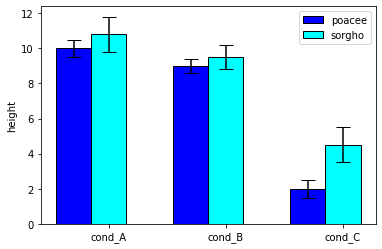Consider that you have several groups, and a set of numerical values for each group. A barplot can be used to represent the average value of each group. However, this kind of figure must be accompanied by error bars showing the confidence interval of each group. This example shows how to draw the confidence interval, but not how to calcultate them. Note that you might loose an information: the distribution of the values is not available in this chart. I strongly advise you to use a boxplot or a violin plot instead.

``````# libraries
import numpy as np
import matplotlib.pyplot as plt

# width of the bars
barWidth = 0.3

# Choose the height of the blue bars
bars1 = [10, 9, 2]

# Choose the height of the cyan bars
bars2 = [10.8, 9.5, 4.5]

# Choose the height of the error bars (bars1)
yer1 = [0.5, 0.4, 0.5]

# Choose the height of the error bars (bars2)
yer2 = [1, 0.7, 1]

# The x position of bars
r1 = np.arange(len(bars1))
r2 = [x + barWidth for x in r1]

# Create blue bars
plt.bar(r1, bars1, width = barWidth, color = 'blue', edgecolor = 'black', yerr=yer1, capsize=7, label='poacee')

# Create cyan bars
plt.bar(r2, bars2, width = barWidth, color = 'cyan', edgecolor = 'black', yerr=yer2, capsize=7, label='sorgho')

# general layout
plt.xticks([r + barWidth for r in range(len(bars1))], ['cond_A', 'cond_B', 'cond_C'])
plt.ylabel('height')
plt.legend()

# Show graphic
plt.show()``````## Contact & Edit

👋 This document is a work by Yan Holtz. You can contribute on github, send me a feedback on twitter or subscribe to the newsletter to know when new examples are published! 🔥